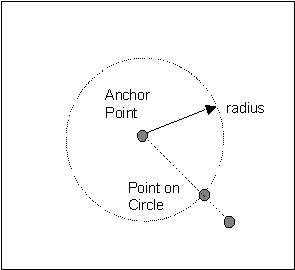This document is archived and information here might be outdated.  Recommended version.

IPoint.ConstrainDistance Method (ArcObjects .NET 10.8 SDK)
 ArcObjects Help for .NET developers > ArcObjects Help for .NET developers > ArcObjects namespaces > Geometry > ESRI.ArcGIS.Geometry > Interfaces > IP > IPoint Interface > IPoint.ConstrainDistance Method
 ArcGIS Developer Help

# IPoint.ConstrainDistance Method

Projects this point to the perimeter of the circle defined by radius and anchor.

```[Visual Basic .NET]
Public Sub ConstrainDistance ( _
ByVal anchor As IPoint _
)
```
```[C#]
public void ConstrainDistance (
IPoint anchor
);
```
```[C++]
HRESULT ConstrainDistance(
IPoint* anchor
);
```
```[C++]
Parameters
constraintRadius is a parameter of type double
anchor
anchor is a parameter of type IPoint*
```

#### Product Availability

Available with ArcGIS Engine, ArcGIS Desktop, and ArcGIS Server.

#### Description

Sets the base Point to a location a specified distance from the input anchor Point along the line between the two points.  ConstrainDistance is used by the editor to fix the distance between an anchor point and an input Point.  Thus, the input to be created must lie on the circumference defined by the anchor point and the fixed distance radius with the angle determined by the user.

#### Remarks

ConstrainDistance[C#]
```private void ConstrainDistance()
{
IPoint point = new PointClass();
point.PutCoords(0, 0);
IPoint newPoint = new PointClass();
newPoint.PutCoords(2,2);
newPoint.ConstrainDistance(Math.PI, point);
System.Windows.Forms.MessageBox.Show(newPoint.X + ", " + newPoint.Y);
```
```}
```
[Visual Basic .NET]

Public Sub ConstrainDistanceTest()
Dim pPoint As ESRI.ArcGIS.Geometry.IPoint
Dim pNPoint As ESRI.ArcGIS.Geometry.IPoint

pPoint = New ESRI.ArcGIS.Geometry.Point
pPoint.PutCoords(0, 0)

pNPoint = New ESRI.ArcGIS.Geometry.Point
pNPoint.PutCoords(2, 2)